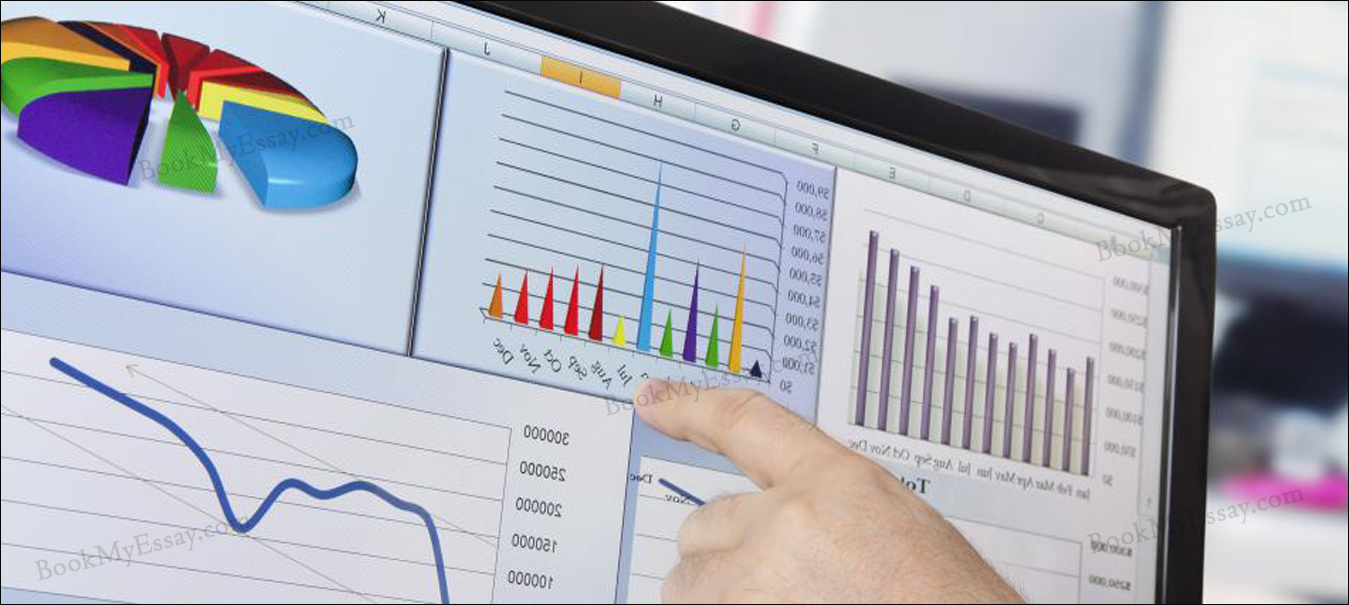Economic analysis and generalizations describe the statements or laws of tendencies in different branches of economics such as consumption, production, exchange and distribution of income. There are some crucial methods of economic analysis which are Deductive method and Inductive method. In the other way, it can be said that economic generalizations usually depicts the statement of uniformities or laws that describes the human behavior in the allocation of the several scarce resources between the alternative ends. With the help of the contents of Economic analysis assignment help, the students can well understand the concept of Economic analysis.## Deductive Method of Economic Analysis

The Deductive method thus explained in the economic generalizations is also called as abstract, a priori method, and analytical. Thus it represents an abstract approach to the derivation of economic generalizations and theories. The primary steps that are involved in the process of deriving economic generalizations through the logic of deductive method are as follows:

• The perception of the problem needs to be enquired into. The analyst or the theorist should have a very clear idea about the problem that they would enquire into. At least the significant variables should be known to them.
• The technical terms need to be defined precisely and the appropriate assumptions should be made which is often called as the premises or postulates. All the technical terms that is used in the analysis as well as for stating the assumptions made clearly, the analyst or theorists need to derive generalizations.
• The deduction of hypothesis which often leads to deriving of the conclusions from the premises through the process of logical reasoning. For deducing hypothesis from the premises taken, one needs to understand hypothesis. It usually explains the relationship between the factors that affect a phenomenon. Through the concept employed in Economic analysis assignment help, the students can clear all the queries regarding Economics analysis.
• Finally the testing of the hypothesis is deduced. Before establishing the hypothesis as generalizations or as principles of Economics, they need to be verified. The Economists usually makes controlled experiments for the verification of the hypothesis as they need to discover the uniformities in the behavioral patterns of the humans.

### Inductive Method of Economic Analysis

The Inductive method of Economic analysis is also referred to as empirical method. Thus it proceeds in deriving the economic generalizations on the basis of observations and experience. Detailed data are collected in this kind of method with regard o a certain economic phenomenon and then to arrive at certain generalizations, some efforts are made that follow from the collected observations. Through the custom assignment writing services, the students can get quality contents from the writers.

For yielding a valid economic generalization, the number of observations is worth to mention. On the basis of extremely few observations, generalizations should not be done. There are three different ways that can be used for deriving the economic principles and theories. Therefore, these three ways are as follows:

• Experimentations
• Observations
• Statistical or Econometric Method